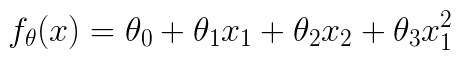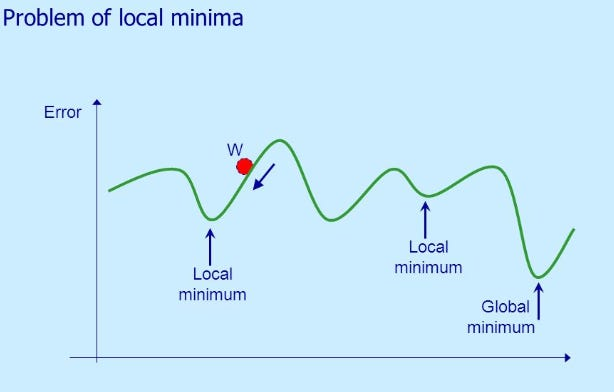# An “Equation-to-Code” Machine Learning Project Walk-Through — Part 3 SGD

Source: Deep Learning on Medium

## Detailed explanation to implement Stochastic Gradient Descent (SGD) and Mini-Batch Gradient Descent from scratch in Python

Hi, everyone! This is “Equation-to-Code” walk-through part 3.

In the previous articles, we talk about in linear separable problem in part 1, and non-linear separable problem in part 2. This time we will implement stochastic gradient descent (SGD) based on the equation.

Part 3 is self-contained. But I won’t give too much explanation for the duplicate content in part 2. If you find something difficult to understand, I recommend reading part 2 first.

Here are the data and code.

The content is structured as follows. `*` means you can skip this step if you already part 2.

1. Preview*
2. Stochastic Gradient Descent (SGD)
3. Mini-Batch Gradient Descent
4. Summary

### 1 Preview

You can skip this step if you already read part 2

First, we look at what we have done in part 2.

Here is the data, non_linear_data.csv

`x1,x2,y0.54508775,2.34541183,00.32769134,13.43066561,04.42748117,14.74150395,02.98189041,-1.81818172,14.02286274,8.90695686,12.26722613,-6.61287392,1-2.66447221,5.05453871,1-1.03482441,-1.95643469,14.06331548,1.70892541,12.89053966,6.07174283,02.26929206,10.59789814,04.68096051,13.01153161,11.27884366,-9.83826738,1-0.1485496,12.99605136,0-0.65113893,10.59417745,03.69145079,3.25209182,1-0.63429623,11.6135625,00.17589959,5.84139826,00.98204409,-9.41271559,1-0.11094911,6.27900499,0`

The data looks like below.

After plotting the data, we found a straight line cannot separate X and O. This kind of problem is called the non-linear separable problem, where data is not linearly separable.

So we introduce polynomial logistic regression, which adds a polynomial term in the linear function.

We use θ to represent the parameter. The θ mark in the left part means the function f(x) has the parameter theta. θ in the right part means there are two parameters. The last term is the polynomial term, which makes the model generalize for non-linear separable data.

Notice that we have x1 and x2 two features in the non_linear_data.csv. We pick up x1 to as the polynomial term. So the function should become below form.a specific form fit to our data

Then we introduce standardization.

• 𝜇 is mean in each column
• 𝜎 is the standard deviation in each column

As for the prediction model, we use the sigmoid function. Below is the vector representation.

We use the z to represent the linear function and pass it to sigmoid function. The sigmoid function will give a probability for each data sample. We have two class in our data, one is `1` and another is `0`.

Alright, we prepared our data, model (sigmoid), and what else do we need? Yes, a goal function. A goal function can guide us on how to update the parameter in the right way. As for the sigmoid (logistic regression), we usually use log likelihood as the goal function. More specifically, we need to calculate the derivative of the log-likelihood function. Here I will directly give the final update equation. (If you are interested in how to get this equation, this video should be helpful)

θj is the j-th parameter.

• η is the learning rate, we set it as 0.001 (1e-3).
• n is the number of data samples, in our case, we have 20.
• i is the i-th data sample

A Numpy array-like version might be easy to understand.

We plot the model line and accuracy line.

Below is the whole code we left after part 2.

If you find something difficult to understand, you could read part 2 for a detailed explanation.

### 2 Stochastic Gradient Descent (SGD)

The main reason we use SGD is to avoid local minima.the parameter is trapped in a local minimum

The basic idea is updating the parameter by randomly selecting one data in each updating. So the parameter is easier to get out of local minimum.

This is the gradient descent form. We can see in order to update θj, we use the whole training data (the Σ part). The code is below.

`# initialize parametertheta = np.random.randn(4)`
`# update parameterfor _ in range(epoch): theta = theta - ETA * np.dot(f(mat_x) - train_y, mat_x)`

But in SGD, we only use one data a time.

Here `k` means the data that we randomly selected.

`# initialize parametertheta = np.random.randn(4)`
`# update parameterfor _ in range(epoch): # sgdp = np.random.permutation(len(mat_x)) for x, y in zip(mat_x[p, :], train_y[p]): theta = theta - ETA * (f(x) - y) * x`
• p contains the random index list of the whole data set, for example, [ 5, 12, 17, 14, 8, 9, 10, 2, 13, 18, 15, 16, 1, 0, 6, 11, 7, 4, 3, 19]
• for loop pick up one data each time to update the parameter theta

You can think SGD as this way. In each epoch, both gradient descent and SGD using the whole data set to update the parameter. Gradient descent update parameter with whole ordered data. But the SGD update parameter with one randomly selected data, which can is easier to get out of local minimum.

The accuracy line. We can see the convergence is faster than gradient descent.

### 3 Mini-Batch Gradient Descent

SGD is good but the computation is not efficient due to updating parameter by one data in each time.

Using whole data will cause local minima problem (gradient descent), and using one data each time is inefficient. That’s why we use mini-batch gradient descent.

Unlike SGD, we could update the parameter with several data samples. Here `K` is an index set which contains `m` randomly selected data samples.

We have 20 data samples, and we set the batch size`m` as 5.

`import math`
`# initialize parametertheta = np.random.randn(4)`
`# batch sizebatch = 5`
`# calculate steps based on batch sizesteps = int(math.ceil(len(train_x)/batch))`
`# update parameterfor _ in range(epoch):p = np.random.permutation(len(mat_x))  shuffle_x = mat_x[p] shuffle_y = train_y[p]`
`for step in range(steps): x = shuffle_x[step:step + batch, :] y = shuffle_y[step:step + batch] theta = theta - ETA * np.dot(f(x) - y, x)`

Notice that we have to shuffle data in each epoch. The calculate is the same as gradient descent by matrix multiplication. If you are interested, you can find detail explanation see part 1 or part 2.

The accuracy line

The convergence speed is the same with gradient descent. But computation is more efficient.

### 4 Summary

In part 3, we talked about how to implement SGD and Mini-Batch Gradient Descent. You can find the whole code below. Leave comments to let me know whether my article is easy to understand. Stay tuned for the final article in this “Equation-to-Code” series about the regularization.